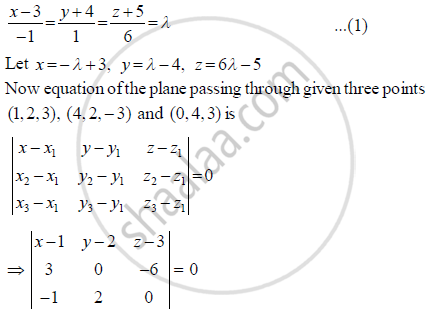Share

# Find the Coordinates of the Point Where the Line Through the Points (3, - 4, - 5) and (2, - 3, 1), Crosses the Plane Determined by the Points (1, 2, 3), (4, 2,- 3) and (0, 4, 3) - CBSE (Commerce) Class 12 - Mathematics

ConceptPlane Equation of a Plane in Normal Form

#### Question

Find the coordinates of the point where the line through the points (3, - 4, - 5) and (2, - 3, 1), crosses the plane determined by the points (1, 2, 3), (4, 2,- 3) and (0, 4, 3)

#### Solution 1

Equation of the line passing through (3, – 4, – 5) and (2, – 3, 1) is⇒ (x - 1)(12) + (y - 2)6 + (z - 3)6 = 0

12x - 12 + 6y - 12 + 6z -18 = 0

12x + 6y + 6z - 42 = 0

2x + y + z - 7 = 0

2(-λ + 3) + 1(λ - 4) + (6λ - 5) - 7 = 0

- 2λ + 6 + λ - 4 + 6λ - 5 -7 = 0

5λ = 10 ⇒ λ = 2

∴ x = -2 +3, y = 2 - 4, z= 12 - 5

∴ x = 1, y = -2, z = 7

∴ Intersection point is (1,-2,7)

#### Solution 2

We know that the cartesian equation of a line passing through two points  (x1, y1, z1)

and (x2, y2, z2)is given by

(x - x_1)/(x_2 - x_1) = (y - y_1)/(y_2 - y_1) = (z - z_1)/(z_2 -  z_1)

So, the equation of a line passing through (3, –4, –5) and (2, –3, 1) is

(x-3)/(2-3) = (y-(-4))/(-3-(-4)) = (z -(-5))/(1-(-5))

=> (x - 3)/(-1) = (y - (-4))/1 = (z-(-5))/6

=> (x - 3)/(-1) = (y+4)/1 = (z+5)/6

Now, the coordinates of any point on this line are given by

(x-3)/(-1) = (y +4)/1 = (z +5)/6 = k

⇒ x = 3- k,y = k - 4, z = 6k - 5, where k is a constant

Let R(3 − kk − 4, 6k − 5) be the required point of intersection.

Now,
Let the equation of a plane passing through (1, 2, 3) be

a(x − 1) + b(y − 2) + c(z − 3) = 0                   .....(1)

Here, abc are the direction ratios of the normal to the plane.

Since the plane (1) passes through (4, 2, −3), so

a(4 - 1) + b(2 - 2) + c(-3 - 3) = 0

⇒ 3a - 6c = 0 .....(2)

Also, the plane (1) passes through (0, 4, 3), so a(0 - 1) + b(4 - 2) + c(3 - 3) = 0

⇒ -a + 2b = 0 .....(3)

Solving (2) and (3) using the method of cross multiplication, we have

a/(0 + 12) = b/(6 - 0) = c/(6 + 0)

=> a/12 = b/6 = c/6

⇒ a/2= b = c = λ (Say)

⇒ a = 2λ, b = λ, c = λ

From (1), we get

2λ(x1λ(y2λ(z3)=0

⇒ 2+7=0                  .....(4)

Putting 3k, k4, 6k5 in (4), we get

⇒ 2(3 - k(k - 4(6k - 5) - 0

5− 10=0

2

Putting k = 2 in R(3 − kk − 4, 6k − 5), we get

R(3 - k, k - 4, 6k - 5R(3 - 2, 2 - 4, × 2 - 5R(1, -2,7)

Thus, the coordinates of the required point are (1, –2, 7) .

Is there an error in this question or solution?

#### APPEARS IN

Solution Find the Coordinates of the Point Where the Line Through the Points (3, - 4, - 5) and (2, - 3, 1), Crosses the Plane Determined by the Points (1, 2, 3), (4, 2,- 3) and (0, 4, 3) Concept: Plane - Equation of a Plane in Normal Form.
S